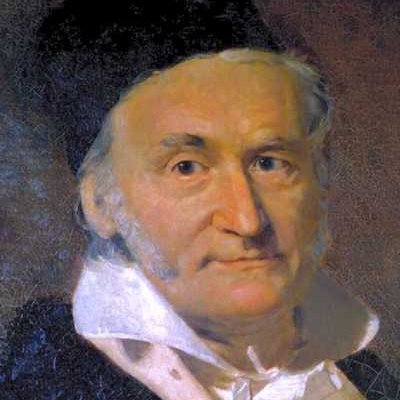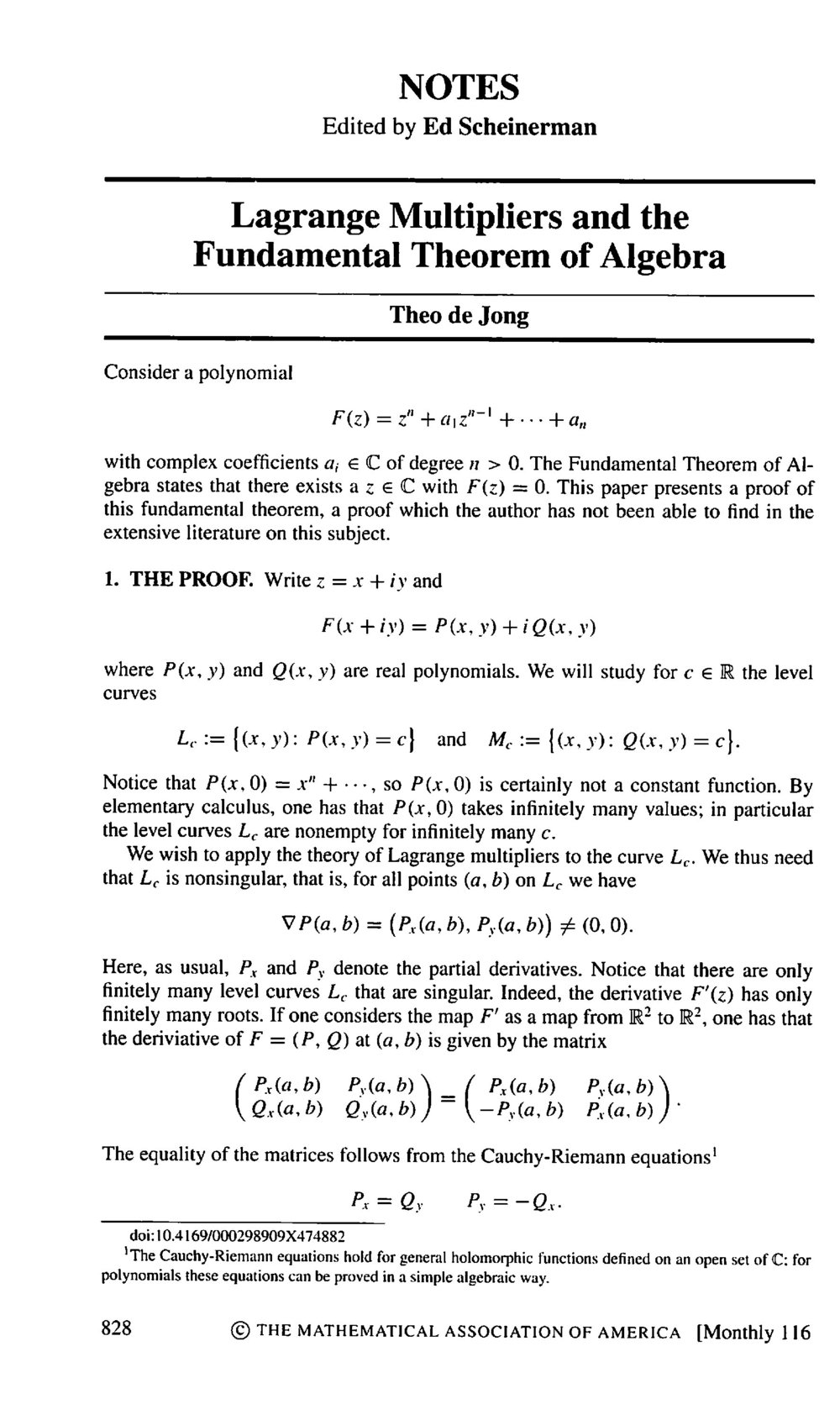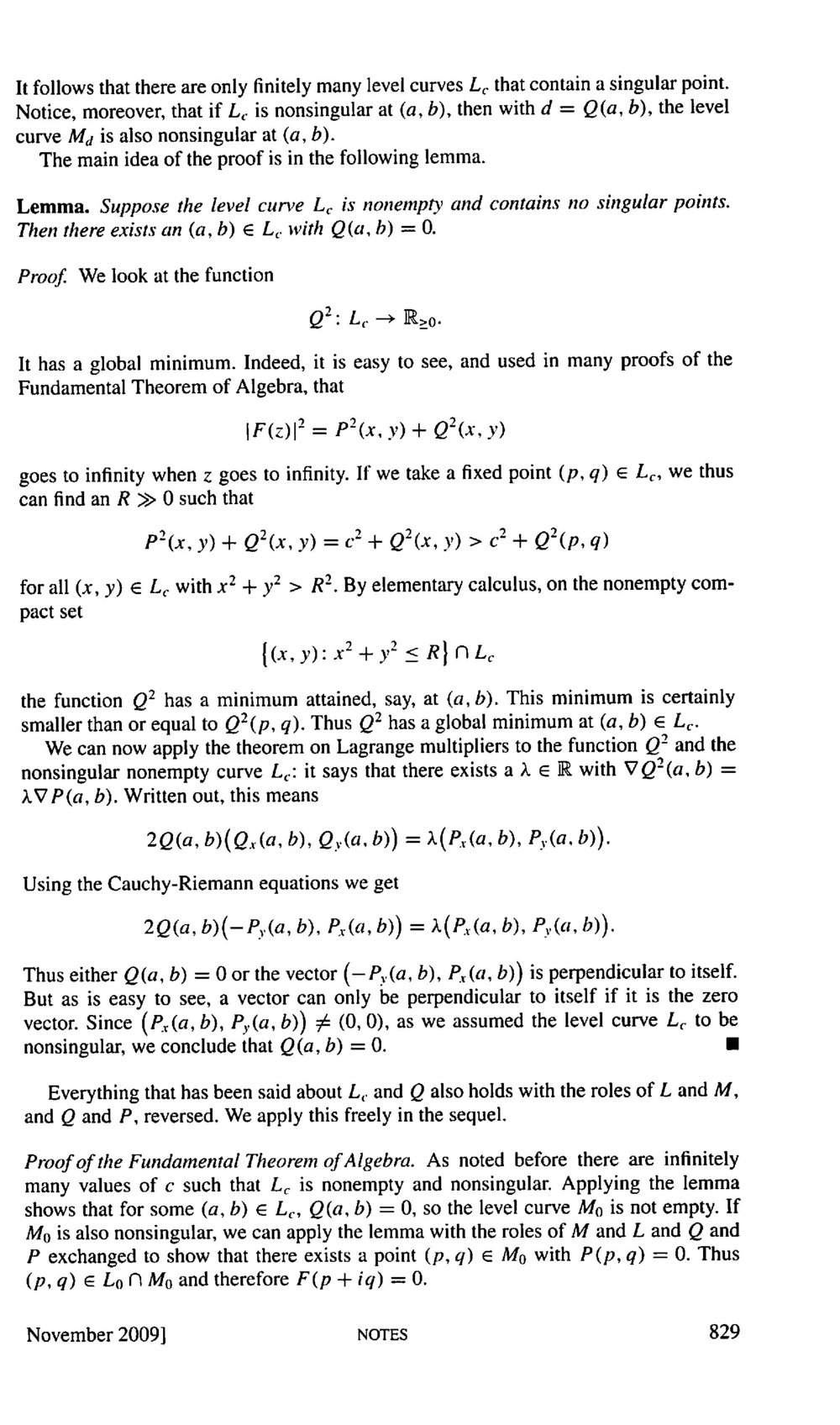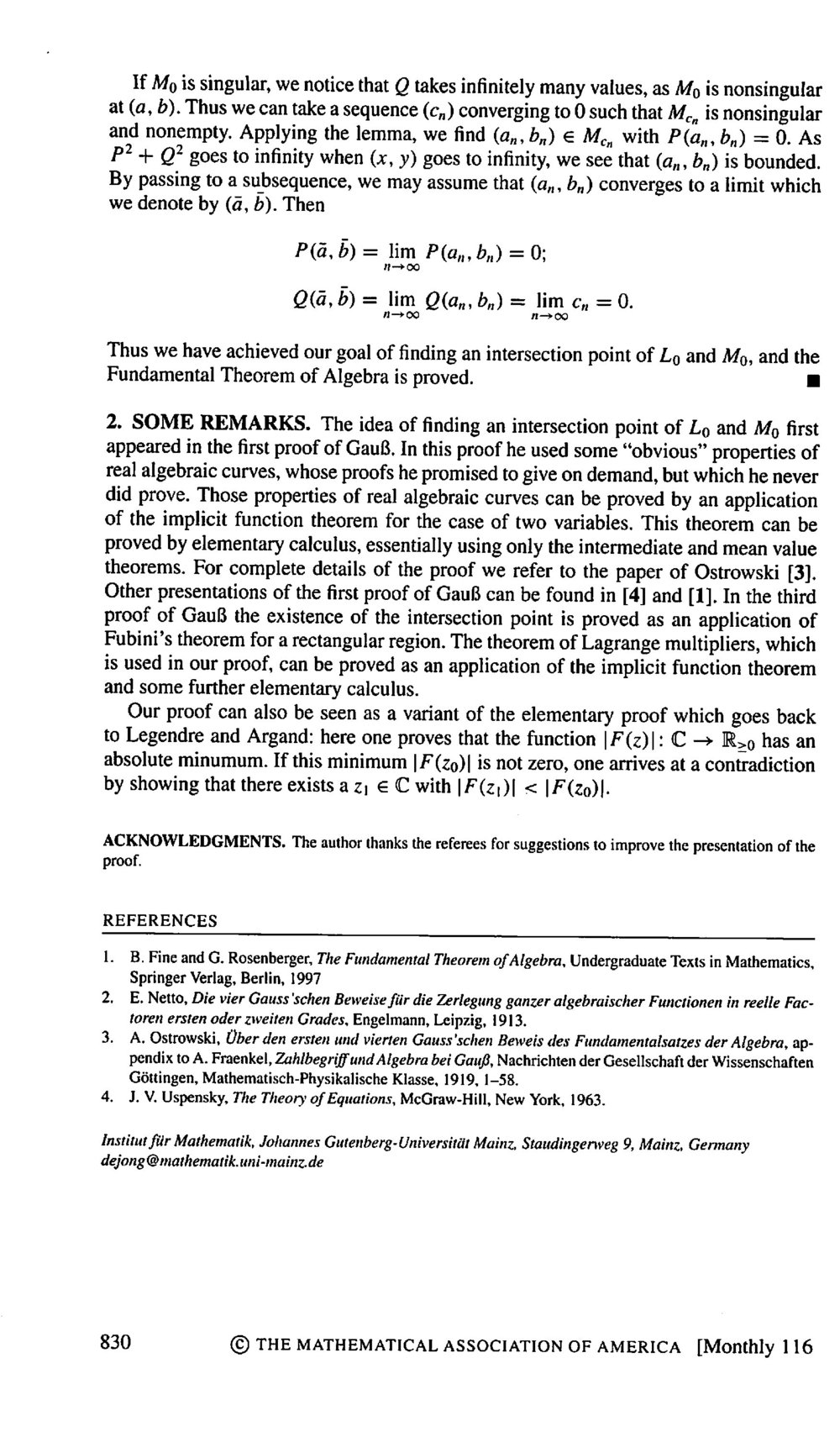6760, Math 21a, Fall 2009
Exhibits page Math 21a 2009, Multivariable Calculus
Lagrange Multipliers and the Fundamental theorem of algebra
Office: SciCtr 434

## Lagrange Multipliers and the Fundamental theorem of algebra

Theo de Jong gives in the Monthly of November 2009 a proof of the fundamental theorem of algebra using the Lagrange multiplier method:

 Theorem: For any complex polynomial F, there is a complex number z=x+i y with ```F(z) = zn + a1 zn-1 + ... + an = 0 . ```

In other words, when writing F(x,y) = P(x,y) + i Q(x,y), there is a point (x,y), where the real functions P and Q are both simultaneously zero. The theorem follows from the following lemma:

 Lemma: If the level curve P= c contains no critical points of P, then it contains a point (a,b) with Q(a,b) = 0.

Proof of the fundamental theorem: There are infinitely many values of c, where the level curve P=c is not empty with no critical points of P. Looking at such a level curve P=c, the lemma implies that the level curve Q=0 is not empty. If Q=0 contains no critical points of Q, the lemma (applied with reversed P and Q) implies that it contains a point (a,b) with P(a,b)=0 proving the theorem. If Q=0 contains a critical point, there is a sequence of points cn converging to 0 for which Q=cn is nonsingular and contains a point (an,bn) with P(an,bn)=0. The sequence (an,bn) is bounded because P2 + Q2 goes to infinity at infinity. There is therefore an accumulation point (a,b). This is the simultaneous root of P and Q.

Proof of the lemma: The function f(x,y) = Q2(x,y) has a global minimum on the contraint g(x,y) = P(x,y) = c because P2 + Q2 goes to infinity for (x,y) to infinity. Such a global minimum satisfies
 the Lagrange equations ``` (fx,fy) = L (gx,gy) g = c ``` which is ``` 2 Q (Qx,Qy) = L ( Px,Py) g = c ```
The Cauchy-Riemann equations Qx = - Py , Qy = Px which hold because F is analytic allow to rewrite this as
```
2 Q (-Py,Px) = L ( Px,Py)
g  = c
```
The first relation tells that either Q=0 or ( Px,Py) is parallel to a vector perpendicular to it, a case not possible because P=c was assumed to have no critical point. There is therefore a point (a,b) on the level curve, where Q(a,b)=0.
Remarks:Image source Read the article below: it gives a bit more details. It mentions also historical contexts like that Gauss was the first using the multivariable idea of looking at level curves of P and Q. The first proof of the fundamental theorem of algebra, I saw myself as an undergraduate was in complex analysis using Liouvilles theorem. In our multivariable calculus/linear algebra sequence, it is rarely taught. I saw Cliff Taubes giving a variant of a Gauss deformation proof once which comes close in simplicity to the above proof. The proof given by de Jong is exciting since it brings the result into the range of calculus for non-math students.